## How to write a code to get following Matrix D?...

```How to write a code to get following Matrix D?```

MY TRY: (But it' s not completely what I seek)

```restart:

k:=1:
M:=2:
N:=2^k*(M+1):

U:=Matrix(M+1,M+1):
for r from 2 to M+1 do
for s from 1 to r-1 do
if type(r+s,odd) then
U(r,s):=2^(k+1)*sqrt((2*r-1)*(2*s-1)):
else 0 end if:
end do:
end do:

#Let's find Matrix D, In here it' s shown matrix DD
DD:=Matrix(N,N,shape=diagonal,fill=U);```

## How to transform a permutation into numbers and vi...

Dear all,

I hope everyone helps me about the problem of converting a permutation into numbers and vice versa on maple

Let Sn denote the set of all permutations of the set {0, 1, ..., n − 1}

Lehmer code is a bijective function l : Sn ->{0, 1, 2, ..., n! − 1}.

Define function l(S)=Sum(ci x (n − 1 − i)! ) where S in Sn and ci is the number of elements of the set { j > i | sj < si }

Inverse Lehmer code is a bijective function l−1 : {0, 1, 2, ..., n! − 1} -> Sn

For example: n=4 -->{0, 1, 2, 3}, for S = (0, 2, 1, 3) , so c0 = 0, c1 = 1 ( j=2 > i=1 and sj = 1 < si = 2), c2 = 0, c3 = 0 -->

I(S) = 0 x (3-0)! + 1 x (3 - 1)! + 0 x (3-2)! + 0 x (3 - 3)! = 2! = 2. So I((0, 2, 1, 3)) =2  and I-1(2) = (0, 2, 1, 3).

## How do I sovle Double integration of function cont...

restart;

##########  omega and theta are variables,where J,F,H,etc are constants.

t1:=-1/(-16.*omega^2+exp(-4*omega)+exp(4*omega)-2.)*(-(0.5817764173e-1*I)*exp((2/9)*omega*cos(theta))*omega^5*cos(theta)*J-(.6981317009*I)*exp((2/9)*omega*cos(theta))*omega^4*cos(theta)*H-0.4524927691e-1*exp(.2222222222*omega*(cos(theta)-9.))*cos(theta)*omega^3*G-.6205615118*exp(.1111111111*omega*(2.*cos(theta)-9.))*cos(theta)*omega^3*H+.6205615118*exp(.1111111111*omega*(2.*cos(theta)-9.))*cos(theta)*omega^3*F+.9308422676*exp(.2222222222*omega*(cos(theta)-9.))*cos(theta)*omega^4*H-.1034269187*exp(.1111111111*omega*(2.*cos(theta)-9.))*cos(theta)*omega^3*G-0.7757018900e-1*exp(.1111111111*omega*(2.*cos(theta)-9.))*cos(theta)*omega^2*G-0.7757018898e-1*exp(.2222222222*omega*(cos(theta)-9.))*cos(theta)*omega^4*J-0.9696273622e-1*exp(.2222222222*omega*(cos(theta)-9.))*cos(theta)*omega^3*J-0.4524927691e-1*exp(.2222222222*omega*(cos(theta)-9.))*cos(theta)*omega^2*J-.2714956613*exp(.2222222222*omega*(cos(theta)-9.))*cos(theta)*omega^2*H-0.7757018898e-1*exp(.2222222222*omega*(cos(theta)-9.))*cos(theta)*omega^4*G+0.8726646261e-1*exp((2/9)*omega*cos(theta))*omega^3*J)*cos((2/9)*omega*sin(theta));

t2:=int(int(t1,omega=0..infinity),theta=0..2*Pi);

## Is there any Maple command to detect whether the d...

Is there any Maple command to detect whether the degree of a polynomial w.r.t. all variables is less or equal 1?

For example, if \$f=ax+by-1\$ when \$x\$ and \$y\$ are variables then the output is true and if \$g=a*x^2+b*y-c\$ then it returns false.

## Maple Cloud problem...

Problem copying a document to Maple Cloud (Public domain)

• Verified email is correct

• Chose File → Save to Cloud... (or clicked Send Document to Cloud on Maple Cloud palette) either way opend Login dialog

• Entered  Account = Maplesoft account, my Email and password then clicked Log in.  The following message appears: "The account type or email address or password is incorrect"

I've saved .mw files to Maple Cloud before with no problems.  Any help appreciated.

Thanks, Les

## Phase portrait ...

I have the equation (𝑑𝑥/𝑑𝑡)^2=𝑎𝑥^3+𝑏𝑥^2+𝑐𝑥+𝑑.

How do I plot 𝑑𝑥/𝑑𝑡 against x? (as the coefficients a, b, c and d vary)

I expect it looks like the following graph: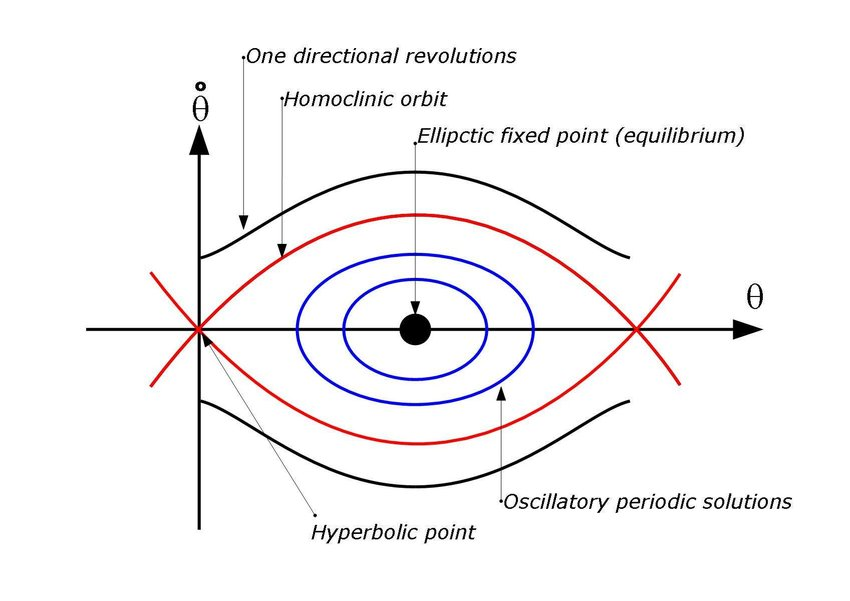## Geodesics on cube...

Hello everybody! I want to build closed geodesic on a cube. I know how to determine the next face, but I do not know how to determine which edge and in what proportion the geodesic will cross.Here is NET for geodesic on cube. Geodesic type (3,9). Yes, 3 and 9 is not relative prime, but geodesic (1,3) not closed.Or did I choose the wrong way to solve the problem? Help pls. Thanks.

## Is there a riemann sum to integral converter?...

Is there a riemann sum to integral converter?

If not then,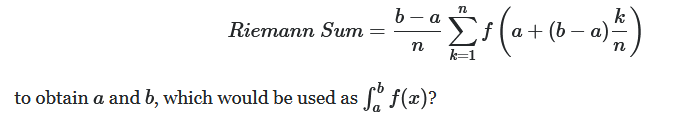So I thought I could make some progress using the maple limit command in the this problem:

limit(Sum(csc(Pi*x/i)^3*sin(Pi*x)^3/i^3, i = 1 .. n), n = infinity)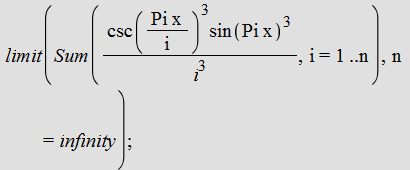which gives signum(sin(Pi*x)^3/x^3)*infinitybut what does this mean?

How do I convert Sum(csc(Pi*x/i)^3*sin(Pi*x)^3/i^3, i = 1 .. n), n = infinity to an integral or from this result?

## Error, invalid input: subs received fsolve...

I'm trying to solve this set of two equations forn where (EQ1 and EQ2 are already défined in fontions of the followings variables (k, wr ,R,Pi), and i'm using the following loop

eqns:={EQ1,EQ2}:

for i from 1 by 1 to 101 do R:=(i-1):S:=fsolve((eqns), {k, wr},{k=0..10,wr=0..10} ):v(i):=(subs(S,(wr))):w(i):=(subs(S,(k)))end do:
Error, invalid input: subs received fsolve({-0.6391108652e160*k+0.2384499927e160*wr+0.714075224e160*k^3-0.4729440685e160*wr^3-0.4025871558e160*k^5+0.1700629083e159*wr^5-0.4813673552e156*k^9+0.1033594302e160*k^7-0.1044324938e156*wr^7-0.1017755535e159*k^3*wr^4+0.2163976160e160*k^5*wr^2-0.7986601863e160*k^4*wr-0.2729449277e160*k^6*wr+0.8693579523e154*k^3*wr^6+0.4453377949e156*k^4*wr^5-0.2636332727e157*k^5*wr^4-0.5817233940e157*k^7*wr^2+0.5719400327e157*k^6*wr^3-0.2875232976e161*k^2*wr+0.2294793648e161*k*wr^2+0.1483050053e158*k^2*wr^5-0.3944893217e159*k^4*wr^3-0.464413477e159*k^2*wr^3+0.2760407324e157*k^8*wr+0.8238787577e156*k*wr^6-0.1081920595e...

i do get a solution for Pi/2 and Pi/3 but beyond this value i get the above error

## Zeros of analytic function...

Dear Sir,

I have a question: I have an analytic function depending on a real parameter that is of the form  F_y (z) with y>0 and z is complex.

I search the zeros  of F_y(z), that is the complex z staisfying F_y(z)=0. I used  the Maple function

RootFinding:-Analytic(F, z, re = -5 .. 0, im = -100 .. 100, );

but he displays me an error message  "Error, (in RootFinding:-Analytic) the function, -(100+I*z)^(1/2)*(80-I*z)^2*cosh((1/150)*(100-I*z)^(1/2)*Pi)*sinh((1/150)*(100+I*z)^(1/2)*Pi)+(100-I*z)^(1/2)*(80+I*z)^2*cosh((1/150)*(100+I*z)^(1/2)*Pi)*sinh((1/150)*(100-I*z)^(1/2)*Pi)+(2*I)*y*z^2*cosh((1/150)*(100-I*z)^(1/2)*Pi)*cosh((1/150)*(100+I*z)^(1/2)*Pi), depends on more than one variable: {y, z}. "

Can you help me to resolve my problem?

Best regards,

Zayd.

## Is it possible to tell Maple NOT to return trivial...

Is there an option or setting to tell Maple not to return trivial solution as a solution to a PDE? Even though trivial solution is correct, it is not something I wanted, and it is making it hard for me to check if the solution is trivial or not.

Here is an example:

```pde:=I*diff(f(x,t),t)=-diff(f(x,t),x\$2)+2*x^2*f(x,t):
bc:=f(-infinity ,t)=0,f(infinity,t)=0:
pdsolve([pde,bc],f(x,t))```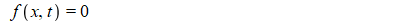Mathematica returns this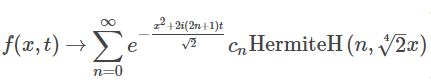If there is no such option in Maple, what would be a good way to check for trivial solution using Maple code?

Just checking if rhs(sol)=0 does not work all the time, as some actual solutions are returned with 0 in rhs, like this example

```pde := diff(u(x, y), x) + u(x,y)*diff(u(x, y),y) =0:
sol:=pdsolve(pde,u(x,y)):
sol:=DEtools:-remove_RootOf(sol)
```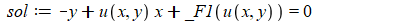So I need either find a way to tell Maple not to return trivial solution, or good robust way to check if solution returned is the trivial solution so I can reject it.

Any suggestion from the Maple experts how to handle this? I am now just interested in result from pdsolve, not dsolve.

The input is the PDE itself, read from a file. So this has to be done without visual inspection on the screen as the program reads the PDE from a file and process it. So the dependent variable and the independent variables are all included in the PDE itself. To make it more clear, I need a function such as

```is_solution_trivial :=proc(pde,sol)
#decide if sol is trivial solution or not.
#one possibility is to find from the PDE the dependent variable
#and the independent variables as in u(x,y,t,....) and then check
#if sol  has the form u(x,y,t,....) =0 ?
end proc;```

The above I can use until I figure how to tell Maple NOT to return trivial solution in first place!

Maple 2019.1

## Solving simultaneous equations and forcing Maple t...

Hi,

I am trying to find p and q from this simultaneous equation as a function of system parameters. I do not know the parameters and I need an expression. But Maple simply just gives p=0 and q=0 as an answer

```Eq1:=61*q*L__1^2*C*e*eta/(16*omega__n^2)+5*q*L__1^2*C*e^3*eta^3/(8*omega__n^4)+3*C*p^3*gamma__1*(1/4)+3*q*C*p^2*R__n/(4*omega__n)+q*L__1^2*C*e^4*eta^4/(16*omega__n^5)+145*q*L__1^2*C/(64*omega__n)+3*q^3*C*R__n/(4*omega__n)+3*p*C*q^2*gamma__1*(1/4)+q*R*C/(4*omega__n)+19*q*L__1^2*C*e^2*eta^2/(8*omega__n^3):
Eq2:=-3*C*p^3*R__n/(4*omega__n)-3*p*C*q^2*R__n/(4*omega__n)-p*L__1^2*C*e^4*eta^4/(16*omega__n^5)-5*p*L__1^2*C*e^3*eta^3/(8*omega__n^4)-19*p*L__1^2*C*e^2*eta^2/(8*omega__n^3)-61*p*L__1^2*C*e*eta/(16*omega__n^2)-145*p*L__1^2*C/(64*omega__n)-p*R*C/(4*omega__n)+3*q*C*p^2*gamma__1*(1/4)+3*q^3*C*gamma__1*(1/4):
sys := { Eq1 , Eq2 };solve( sys, {p,q} );
```

Is there any way to help Maple to try other conditions, I know the only solution should not be just p=0 and q=0.

Thanks,

Baharm31

## "How to solve equation?"...

Hi everyone.I need to know what is "t" in this equation.

(1+0.25cost)×(cos7t)=0.1

I dont need numeric solution.I need step by step solution.

Thanks.

## Unapply versus arrow operator...

Hi,

I do not really understand the difference between annrow operator and unapply.
From the help pages it seems that unapply "creates" an arrow operator and thus that they could be two different ways to do the same thing.

restart:

f := x+y:

a := indets(f):                  # just because f can be more complex than the f above
g := (op(a)) -> f;              # generates an error, "operators not of a symbol type"
h := unapply(f, (op(a)))   # ok, but with a strange output
h := (x__1, y__1) -> x__1+y__1

So it seems that Maple has transform by itself the indexed x and y into symbols x__1 and y__1.

Could you explain me what happened exactly ?

TIA

## How to tell Maple to give the highest order of der...

`f := 2*y(t)*(diff(x(t), t))^2+3*(diff(x(t), t\$3))-3*x(t)*(diff(y(t), t));`